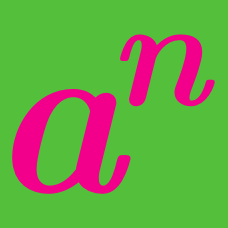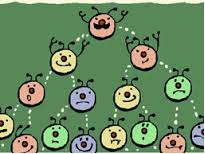Algebra

# Exponential Functions: Level 1 Challenges

$\large \color{#624F41}5^{55} + \color{#624F41}5^{55} + \color{#624F41}5^{55} + \color{#624F41}5^{55} +\color{#624F41} 5^{55} = \, ?$A scientist has a jar of bacteria which double every minute. After one hour, she sees that the jar is full of bacteria. After how many minutes was the jar half full?

$\large \frac {\color{#D61F06}6^{\color{#3D99F6}{2007}} - \color{#D61F06}6^{\color{#3D99F6}{2006}}}{\color{#20A900}{30}} = \ ?$

$\large 2^x=\dfrac{1}{2^y}, \quad\quad\quad x + y = \ ?$

$\large 7^{x+1} + 7^{x-1} = 50, \ \ \ \ \ x = \ ?$

×